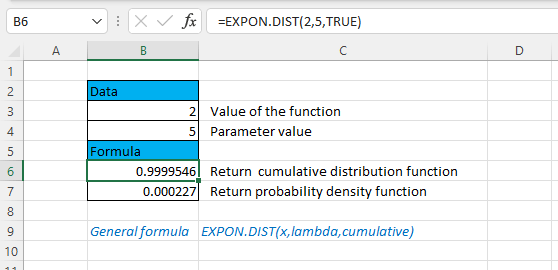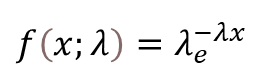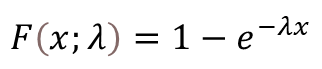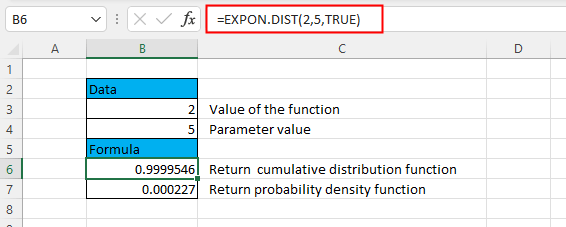## Excel EXPON.DIST Function### Description

The Excel EXPON.DIST function returns the exponential distribution based on the given x value and the parameter of the distribution.

### Syntax and arguments

Formula syntax

EXPON.DIST(array1,array2,cumulative)

Arguments

 X: Required, the value of the function. It must be ≥ 0. Lambda: Required, the parameter of the distribution. It must be ≥ 0. Cumulative: Required, a logical argument that specifies the type of the exponential function to be returned.TRUE: returns the cumulative distribution function;FALSE: returns the probability density function.

### Remarks

1. The function returns #VALUE! error value if either argument x or lambda is a non-numeric value.

2. The function returns #NUM! error value if either argument x < 0 or lambda ≤ 0.

3. The equation for the probability density function is:4. The equation for the cumulative distribution function is:### Version

Excel 2010 and later versions.

### Usage and Examples

Basic usage

For instance, the argument x is 2 and the argument lambda is 5, to get the relative cumulative distribution function and probability density function, please use the formula as these:

=EXPON.DIST(2,5,TRUE)  //Return cumulative distribution function

=EXPON.DIST(2,5,FALSE)  //Return probability density function

Press Enter key.#### Other Functions:

• Excel COVARIANCE.P Function
The Excel COVARIANCE.P function returns the population variance of two data sets which is used to determine the relationship between two data sets.

• Excel NORM.S.DIST Function
The NORM.S.DIST calculates and returns the standard normal cumulative distribution function or probability density function of a value for an arithmetic mean of 0 and standard deviation of 1

• Excel NORM.S.INV Function
The NORM.S.INV calculates and returns the inverse of the standard normal cumulative distribution that has an arithmetic mean of 0 and standard deviation of 1 with a given probability.

### The Best Office Productivity Tools

#### Kutools for Excel - Helps You To Stand Out From Crowd

 Popular Features: Find, Highlight or Identify Duplicates  |  Delete Blank Rows  |  Combine Columns or Cells without Losing Data  |  Round without Formula ... Super VLookup: Multiple Criteria  |  Multiple Value  |  Across Multi-Sheets  |  Fuzzy Lookup... Adv. Drop-down List: Easy Drop Down List  |  Dependent Drop Down List  |  Multi-select Drop Down List... Column Manager: Add a Specific Number of Columns  |  Move Columns  |  Toggle Visibility Status of Hidden Columns  |  Compare Columns to Select Same & Different Cells ... Featured Features: Grid Focus  |  Design View  |  Big Formula Bar  |  Workbook & Sheet Manager | Resource Library (Auto Text)  |  Date Picker  |  Combine Worksheets  |  Encrypt/Decrypt Cells  |  Send Emails by List  |  Super Filter  |  Special Filter (filter bold/italic/strikethrough...) ... Top 15 Toolsets:  12 Text Tools (Add Text, Remove Characters ...)  |  50+ Chart Types (Gantt Chart ...)  |  40+ Practical Formulas (Calculate age based on birthday ...)  |  19 Insertion Tools (Insert QR Code, Insert Picture from Path ...)  |  12 Conversion Tools (Numbers to Words, Currency Conversion ...)  |  7 Merge & Split Tools (Advanced Combine Rows, Split Excel Cells ...)  |  ... and more

Kutools for Excel Boasts Over 300 Features, Ensuring That What You Need is Just A Click Away...#### Office Tab - Enable Tabbed Reading and Editing in Microsoft Office (include Excel)

• One second to switch between dozens of open documents!
• Reduce hundreds of mouse clicks for you every day, say goodbye to mouse hand.
• Increases your productivity by 50% when viewing and editing multiple documents.
• Brings Efficient Tabs to Office (include Excel), Just Like Chrome, Edge and Firefox.